Solving a picross puzzle

Now that you know the goal and the game rules of hanjie, we are going to learn how to solve a picross puzzle through this tutorial.

Discover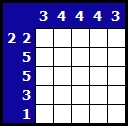First of all here is what a picross puzzle looks like.
Here we have a 5 by 5 puzzle. There are several numbers on the left side and above the puzzle. Each sequence of numbers in front of a line or above a column indicates how many squares have to be blackend in this line or columns.
For instance, the 3 above the first column indicates that 3 squares have to be blackened, one after the other.

Step one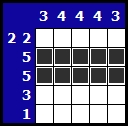First we will blacken the easiest squares.
Take a look at the two 5 on the left side of the puzzle. The indicate that you must blacken five squares on each line. As the puzzle is 5 by 5 squares, you have to blacken each square of those two lines!

Full line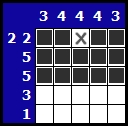Now take a look at the first line. The number sequence is 2 2, that means that there are two series of two squares, those series being separated by AT LEAST one empty square.
Let's do some maths: we have 2+1+2 (at least one empty square between the two series) = 5 squares (it's a minimum). This line being exactly 5 squares long, there is only one solution: 2 black squares at the beginning of the line and 2 black squares at the end.

Column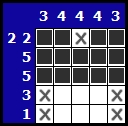Let's deal with columns!
We have 2 columns with a 3 on top. Those columns already have three black squares. Thus, those columns are good and we can check the other squares in it.

Beginning of a column / line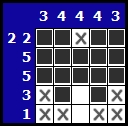Then we have 2 columns with a 4 and three black square in each one. We just need to blacken the fourth square to find the solution for those columns.

Solving the puzzle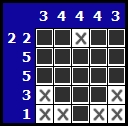Now you can take care of the last squares!

Congratulations! You just solved your first picross puzzle! Now you can try to solve as much picross puzzles as you can!